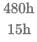Humans and robots can both perform a job but at different efficiencies. Fifteen humans and five robots working together take thirty days to finish the job, whereas five humans and fifteen robots working together take sixty days to finish it. How many days will fifteen humans working together (without any robot) take to finish it?
• a)
45
• b)
36
• c)
32
• d)
40

### Related TestJai Bhagwan May 22, 2020Let the efficiency of humans be 'h' and the efficiency of robots be 'r'.
In the first case, Total work = (15h + 5r) * 30......(i)
In the second case,
Total work = (5h + 15r) * 60......(ii)
On equating (i) and (ii), we get (15h + 5r) * 30 = (5h + 15r) * 60
Or, 15h + 5r = 10h + 30r
Or, 5h = 25r
Or, h = 5r
Total work = (15h + 5r) * 30 = (15h + h) * 30 = 480h Time taken by 15 humans =days= 32 days.
Hence, option C is the correct answer.

Let the efficiency of humans be h and the efficiency of robots be r.In the first case, Total work = (15h + 5r) * 30......(i)In the second case,Total work = (5h + 15r) * 60......(ii)On equating (i) and (ii), we get (15h + 5r) * 30 = (5h + 15r) * 60Or, 15h + 5r = 10h + 30rOr, 5h = 25rOr, h = 5rTotal work = (15h + 5r) * 30 = (15h + h) * 30 = 480h Time taken by 15 humans = days= 32 days.Hence, option C is the correct answer.
Do you know? How Toppers prepare for CAT Exam
With help of the best CAT teachers & toppers, We have prepared a guide for student who are preparing for CAT : 15 Steps to clear CAT Exam